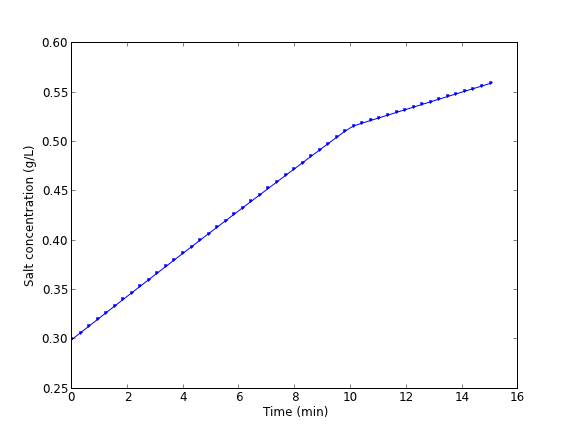ODEs with discontinuous forcing functions

| categories: ode | tags: | View Comments

A mixing tank initially contains 300 g of salt mixed into 1000 L of water. At t=0 min, a solution of 4 g/L salt enters the tank at 6 L/min. At t=10 min, the solution is changed to 2 g/L salt, still entering at 6 L/min. The tank is well stirred, and the tank solution leaves at a rate of 6 L/min. Plot the concentration of salt (g/L) in the tank as a function of time.

A mass balance on the salt in the tank leads to this differential equation: $$\frac{dM_S}{dt} = \nu C_{S,in}(t) - \nu M_S/V$$ with the initial condition that $$M_S(t=0)=300$$. The wrinkle is that the inlet conditions are not constant.

$$C_{S,in}(t) = \begin{array}{ll} 0 & t \le 0, \\ 4 & 0 < t \le 10, \\ 2 & t > 10. \end{array}$$

import numpy as np
from scipy.integrate import odeint
import matplotlib.pyplot as plt

V = 1000.0 # L
nu = 6.0  # L/min

def Cs_in(t):
'inlet concentration'
if t < 0:
Cs = 0.0 # g/L
elif (t > 0) and (t <= 10):
Cs = 4.0
else:
Cs = 2.0
return Cs

def mass_balance(Ms, t):
'$\frac{dM_S}{dt} = \nu C_{S,in}(t) - \nu M_S/V$'
dMsdt = nu * Cs_in(t) - nu * Ms / V
return dMsdt

tspan = np.linspace(0.0, 15.0, 50)

M0 = 300.0 # gm salt
Ms = odeint(mass_balance, M0, tspan)

plt.plot(tspan, Ms/V, 'b.-')
plt.xlabel('Time (min)')
plt.ylabel('Salt concentration (g/L)')
plt.savefig('images/ode-discont.png')You can see the discontinuity in the salt concentration at 10 minutes due to the discontinous change in the entering salt concentration.